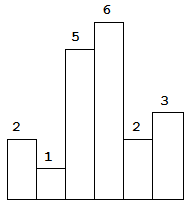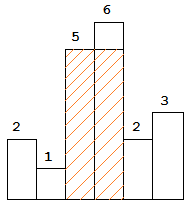## 84. 柱状图中最大的矩形

2019-02-12 13:54:03 by Sikoay with 0 comments 1 Hits## 问题：```输入: [2,1,5,6,2,3]

## 解答：

class Solution {
function largestRectangleArea(\$heights) {
\$max_area = 0;
for(\$i = 0;\$i < count(\$heights);\$i++){
\$height = \$heights[\$i];
\$width = 1;

if(\$height == 0){
continue;
}
for(\$n = \$i-1;\$n >= 0;\$n--){
if(\$heights[\$n] > \$height){
\$width++;
}else if (\$heights[\$n] == \$height){
continue 2;
}else{
break 1;
}
}
for(\$m = \$i+1;\$m < count(\$heights);\$m++){
if(\$heights[\$m] >= \$height){
\$width++;
}else{
break;
}
}
if(\$max_area < \$width * \$height){
\$max_area = \$width * \$height;
}
}
return \$max_area;
}
}

Tags: